# Texas Go Math Grade 1 Lesson 20.4 Answer Key Give to Charity

Refer to our Texas Go Math Grade 1 Answer Key Pdf to score good marks in the exams. Test yourself by practicing the problems from Texas Go Math Grade 1 Lesson 20.4 Answer Key Give to Charity.

## Texas Go Math Grade 1 Lesson 20.4 Answer Key Give to Charity

Explore

Draw to show a way you can share with others.

FOR THE TEACHER • Have children discuss ways they can help and share with others. Then have them draw to show one way they share.

Math Talk
Mathematical Processes

What can you do to share with others?

Model and Draw

Rachel’s family shares some of the Giving t0 or sharing with money they earn with others. Rachel others in need is charity, puts some coins to share in a jar.
Giving to or sharing with others in need is charity.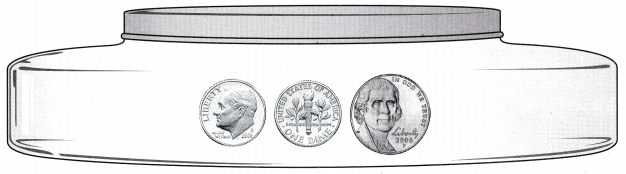What is the total value of the coins?
The value of total coins are 1 dime + 1 dime + 1 nickel
1 dime = 10¢
1 nickel = 5¢
The total value is 10¢ + 5¢ = 15¢.

Share and Show

Answer the question. You may use coins.

Question 1.
Cindi puts these coins in the sharing jar. What is the total value of these coins?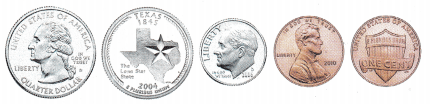Given that
Cindi puts her coins in the Sharing jar.
The total value of coins are 1 quarter + 1 penny + 1 dime + 1 dime + 1 cent.
1 quarter = 25¢
1 Penny = 1 ¢
1 dime = 10 ¢
The total value is 25¢ + 1¢ + 10¢ + 1¢ = 37¢.

Question 2.
Ava puts 1 quarter, 4 dimes, and 5 pennies in the sharing jar. What is the total value of these coins? Draw and label the coins you use.
Ava puts 1 quarter, 4 dimes and 5 pennies in the Sharing jar.
The total value of coins are
1 quarter = 25¢
1 dime = 10¢ then 4 dime = 4 × 10 = 40¢
1 Penny = 1 cent then 5 pennies = 5 cents
The total value is 25¢ + 40¢ + 5 cents = 70cents.

Problem Solving

Answer the question. You may use coins.Question 3.
Nina puts these coins in the sharing jar. What is the total value of these coins?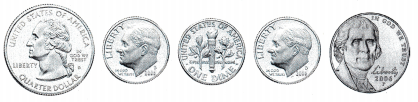The total value of these coins are 1 quarter + dime + dime + dime + nickel =
25 cents + 10 cents + 10 cents + 10 cents + 5 cents = 60 cents.

Question 4.
Liz puts 3 quarters, 1 dime, and 1 nickel in the sharing jar. What is the total value of these coins?
Given that
Liz puts 3 quarters, 1 dime, and 1 nickel in the sharing jar.
The total value of these coins are
1 quarter = 25 cents then 3 quarters = 25 cents × 3 = 75 cents.
1 dime = 10 cents
1 Nickel = 5 cents
Total value of these coins = 75 cents + 10 cents + 5 cents = 90 cents.

Question 5.

H.O.T. Ross has 1 quarter, 3 dimes, and 2 pennies. He puts one coin in the sharing jar. The total value of the coins he has left is 47¢. Which coin did Ross put in the jar?Given that
Ross has 1 quarter, 3 dimes, and 2 pennies
1 quarter = 25 cents
1 dime = 10 cents then 3 dimes = 10 × 3 = 30 cents
1 Penny = 1 cent. then 2 pennies = 2 cents.
Total coins = 25 cents + 30 cents + 2 cents
Total coins = 57 cents
The total value of coins he left is 47¢.
So, 57 cents – 1 dime = 47¢.
The coin did Ross put in the jar = 1 dime

Question 6.

H.O.T. Multi-Step Glenn has 3 quarters and 2 dimes. He puts 1 quarter and 1 dime in the sharing jar. What is the total value of the coins he has left?
Given that
Glenn has 3 quarters and 2 dimes.
He puts 1 quarter and 1 dime in the sharing jar.
Glenn left = 3 quarters – 1 quarter = 2 quarters
2 dimes – 1 dime = 1 dime
2 quarters and 1 dime in cents are
1 quarter = 25 cents then 2 quarters = 2 × 25 = 50 cents.
1 dime = 10 cents
Total he left = 50 cents + 10 cents = 60 cents.

Choose the correct answer. You may use coins.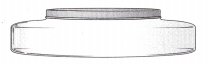Question 7.
Mel puts these coins in the sharing jar. What is the total value of these coins?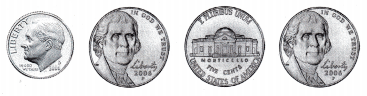(A) 40¢
(B) 25¢
(C) 30¢
The total value of these coins are 1 dime + 1 nickel + 5 cents + 1 nickel = 10 cents + 5 cents + 5 cents + 5 cents = 25 cents
Option B is the correct answer.

Question 8.
Apply Raul puts 1 quarter, 2 nickels, and 3 pennies in the sharing jar. What is the total value of these coins?
(A) 38¢
(B) 48¢
(C) 30¢
Raul puts 1 quarter, 2 nickels and 3 pennies in the sharing box.
The value of coins are
1 quarter = 25 cents
1 nickels = 5 cents then 2 nickels = 2 × 5 = 10 cents
3 pennies = 3 cents
The total value of coins = 25 cents + 10 cents + 3 cents = 38 cents.
Option A is the correct answer.

Question 9.
Multi-Step Lola has 3 quarters, 1 nickel, and 1 penny. She puts 1 quarter in the sharing jar. What is the total value of the coins Lola has left?
(A) 81¢
(B) 86¢
(C) 56¢
Given that
Lola has 3 quarters, 1 nickel and 1 penny.
She puts 1 quarter in the Sharing jar.
The total value of coins that Lola left = 2 quarters, 1 nickel and 1 penny
1 quarter = 25 cents then 2 quarters = 2 × 50 cents.
1 nickel = 5 cents
1 Penny =1 cent
The total value of coins that Lola left = 50 cents + 5 cents + 1 cent = 56 cents.

Question 10.
Texas Test Prep Adan puts 4 dimes in the sharing jar on Monday. He puts 2 dimes and I nickel in the jar on Tuesday. What is the total value of the coins Adan puts in the jar?
(A) 70¢
(B) 75¢
(C) 65¢
Given that
Adan puts 4 dimes in the sharing jar on Monday.
She puts 2 dimes and 1 nickel in the Sharing box on Tuesday
The total value of coins are 6 dimes and 1 nickel
1 dime = 10 cents then 6 dimes = 6 × 10 = 60 cents.
1 nickel = 5 cents.
The total value of the coins are 60 cents + 5 cents = 65 cents.

### Texas Go Math Grade 1 Lesson 20.4 Homework and Practice Answer Key

Answer the question. You may use coins.Question 1.
Dee puts these coins in the sharing jar. What is the total value of these coins?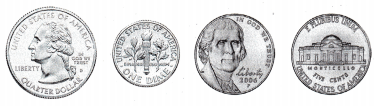The total value of these coins are 1 quarter + 1 dime + 1 nickel + 5 cents = 25 cents + 10 cents + 5 cents + 5 cents = 45 cents.

Question 2.
Sherri puts 2 quarters, 1 dime, and 2 pennies in the sharing jar. What is the total value of these coins?
Given that
Sherri puts 2 quarters, 1 dime and 2 pennies in the Sharing jar.
The total value of these coins are
1 quarter = 25 cents then 2 quarters = 2 × 25 cents = 50 cents
1 dime = 10 cents
2 pennies = 2 cents
The total value of these coins = 50 cents + 10 cents + 2 cents = 62 cents.

Problem Solving

Answer the question. You may use coins.

Question 3.
Multi-Step Marissa has 2 quarters and 3 dimes. She puts 1 quarter and 2 dimes in the sharing jar. What is the total value of the coins she has left?
Given that
Marissa has 2 quarters and 3 dimes.
She puts 1 quarter and 2 dimes in the Sharing jar.
The total value of coins that she left = 1 quarter and 1 dime.
1 quarter = 25 cents
1 dime = 10 cents
The total value of coins that she left = 25 cents + 10 cents = 35 cents.

Lesson Check

Choose the correct answer. You may use coins.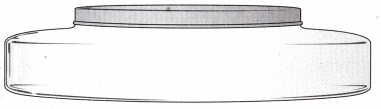Question 4.
Vicki puts these coins in the sharing jar. What is the total value of these coins?(A) 85¢
(B) 80¢
(C) 75¢
The total value of these coins are 1 quarter + 1 quarter + 1 dime + 1 dime + 1 nickel + 5 cents = 25 cents + 25 cents + 10 cents + 10 cents + 5 cents + 5 cents = 80 cents
Option B is the correct answer.

Question 5.
Sean puts 3 dimes, 3 nickels, and 6 pennies in the sharing jar. What is the total value of these coins?
(A) 51¢
(B) 66¢
(C) 61¢
Sean puts 3 dimes ,3 nickels and 6 pennies in the Sharing jar
The value of coins are
1 dime = 10 cents then 3 dimes = 2 × 10 = 30 cents.
1 nickel = 5 cents then 3 nickels = 5 × 3 = 15 cents.
1 Penny = 1 cent then 6 pennies = 6 cents
The total value of these cents are 30 cents + 15 cents + 6 cents = 51 cents.

Question 6.
Ali puts 1 quarter in the sharing jar on Monday. She puts 3 dimes and 2 pennies in the jar on Tuesday. What is the total value of the coins Ali puts in the jar?
(A) 55¢
(B) 57¢
(C) 52¢
Given that,
Ali puts 1 quarter in the sharing jar on Monday.
Ali puts 3 dimes and 2 pennies in the jar on Tuesday.
The total value of coins are 1 quarter, 3 dimes and 2 pennies
1 quarter = 25 cents
1 dime = 10 cents and 3 dimes = 3 × 10 = 30 cents.
1 Penny = 1 cent then 2 penny = 2cents.
Total value of coins = 25 cents + 30 cents + 2 cents = 57 cents.
Option B is the correct answer.

Question 7.
Multi-Step Jimmy has 1 quarter, 3 nickels, and 4 pennies. He puts 3 nickels and 2 pennies in the sharing jar. What is the total value of the coins Jimmy has left?
(A) 25¢
(B) 44¢
(C) 27¢